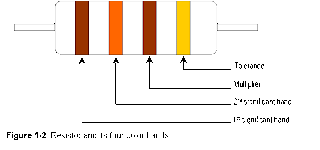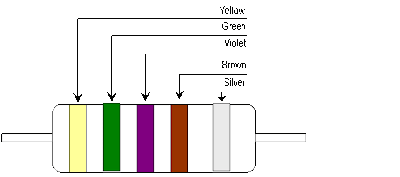## Resistor Color Codes

Resistors may contain three to five color bands. Resistors with +20% tolerances contain only three color bands. Four color bands are usually printed on the body of most resistors. For a resistor with four color bands, the first color represents the first significant figure. The second color band represents the second significant figure. The third color band is the multiplier and the last color band is the tolerance. Below are the lists of colors with their corresponding values when used as significant figure, as multiplier and as tolerance.Example 1. Assume a resistor has color bands of blue, orange, brown and gold (see figure 1-3). The first two bands represents the number 6 and 3 (blue is 6 and orange is 3). The third band is brown, represented by 10, which means that the number 63, the first and second significant figure, should be multiplied by 10. The fourth band is the tolerance. The tolerance in this example is gold represented by + 5%. The resistance value of this resistor is 630 ohms plus or minus 5 percent.Figure 1-3     6     3     x10     =630Ω +10%

Example 2. The resistor with 5 color bands (see figure 1-4) contains the following color.
First band = yellow
Second band = green
Third band = violet
Fourth band = brown
Fifth band = silver

The fifth band which is silver indicates that this resistor has a tolerance of + 10%. The first three bands represents the first, second and third significant figure. Yellow is 4, green is 5 and violet is 7, forming a number value of 457. The fourth band is brown, which means that the first three numbers, 457, will be multiplied by 10. The value of this resistor is 4570Ω + 10%.Figure 1-4     4     5     7     x10    =     4570Ω + 10%